# Polynomials

## Objective

Identify features of polynomial functions including end behavior, intervals where the function is positive or negative, and domain and range of function.

## Common Core Standards

### Core Standards

?

• F.BF.B.3 — Identify the effect on the graph of replacing f(x) by f(x) + k, k f(x), f(kx), and f(x + k) for specific values of k (both positive and negative); find the value of k given the graphs. Experiment with cases and illustrate an explanation of the effects on the graph using technology. Include recognizing even and odd functions from their graphs and algebraic expressions for them.

• F.IF.B.4 — For a function that models a relationship between two quantities, interpret key features of graphs and tables in terms of the quantities, and sketch graphs showing key features given a verbal description of the relationship. Key features include: intercepts; intervals where the function is increasing, decreasing, positive, or negative; relative maximums and minimums; symmetries; end behavior; and periodicity. Modeling is best interpreted not as a collection of isolated topics but in relation to other standards. Making mathematical models is a Standard for Mathematical Practice, and specific modeling standards appear throughout the high school standards indicated by a star symbol (★). The star symbol sometimes appears on the heading for a group of standards; in that case, it should be understood to apply to all standards in that group.

• F.IF.B.6 — Calculate and interpret the average rate of change of a function (presented symbolically or as a table) over a specified interval. Estimate the rate of change from a graph. Modeling is best interpreted not as a collection of isolated topics but in relation to other standards. Making mathematical models is a Standard for Mathematical Practice, and specific modeling standards appear throughout the high school standards indicated by a star symbol (★). The star symbol sometimes appears on the heading for a group of standards; in that case, it should be understood to apply to all standards in that group.

?

• F.IF.B.4

## Criteria for Success

?

1. Describe end behavior as the value of y the function approaches as the value of x either increases to infinity or decreases to negative infinity.
2. Describe how the shape of the function, domain of the function, and end behavior change based on whether the degree of the function is odd or even.
3. Describe the effect the leading coefficient has on the shape of the function and the end behavior of the function.
4. Explain that when all coefficients and terms are unchanged except the degree of the polynomial, the rate of change over a fixed interval will increase with an increasing degree.

## Anchor Problems

?

### Problem 1

What do all of these functions have in common? What's different?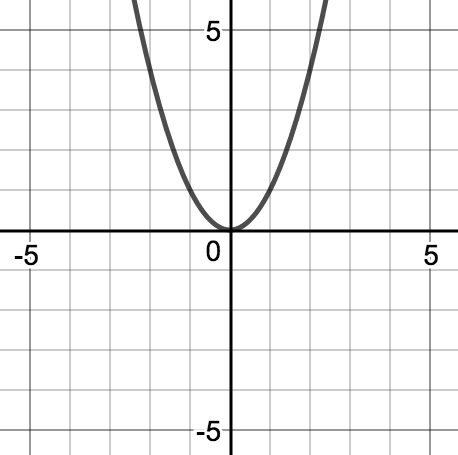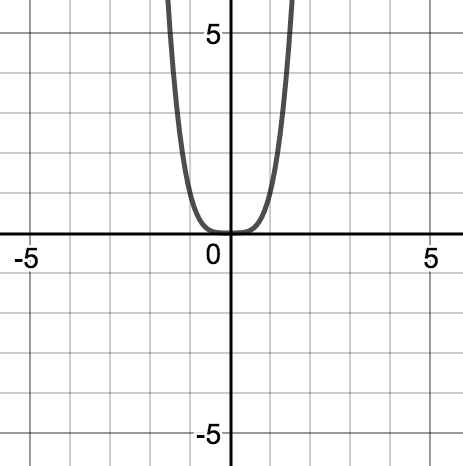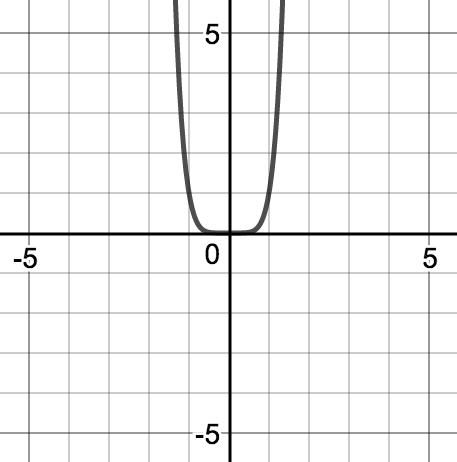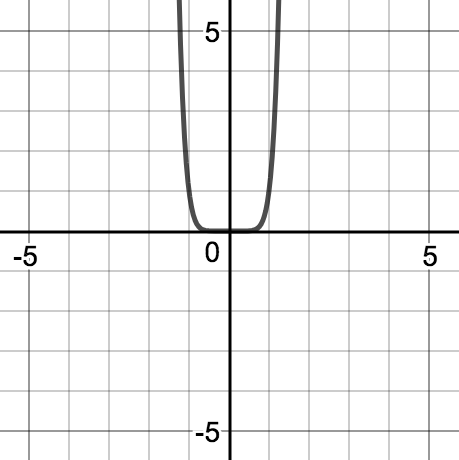${h(x)=x^2}$ ${k(x)=x^4}$ ${l(x)=x^6}$ ${r(x)=x^8}$

What do all of these functions have in common? What's different?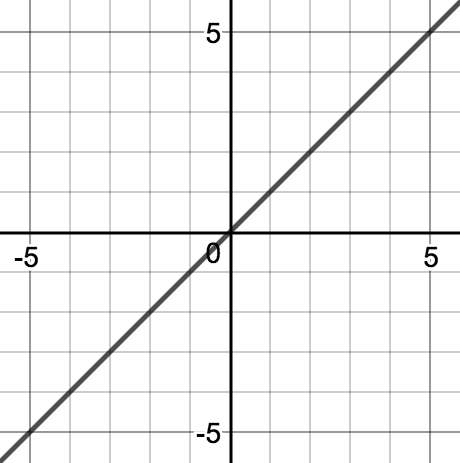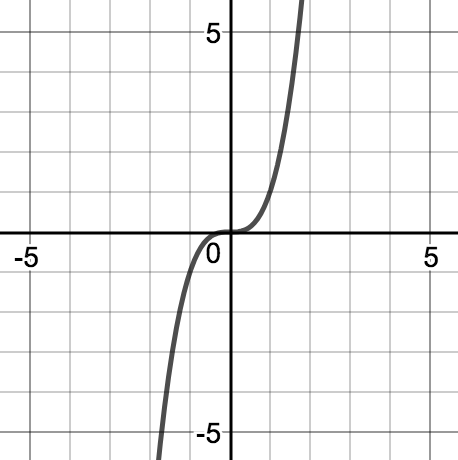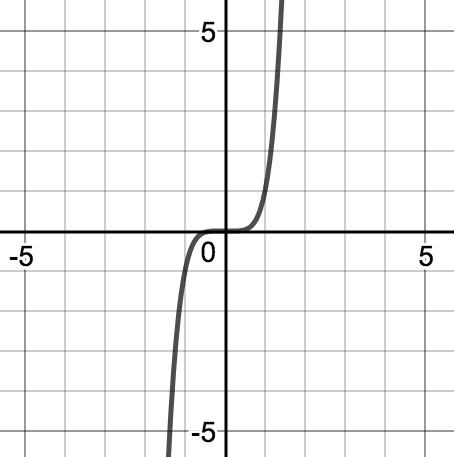${t(x)=x}$ ${j(x)=x^3}$ ${m(x)=x^5}$ ${w(x)=x^7}$

### Problem 2

Write a function and draw a sketch that meets the constraints below.

• Your function tends toward negative infinity when $x$ is very small and tends toward positive infinity when $x$ is very large.
• Your function has the marked points as $x$-intercepts.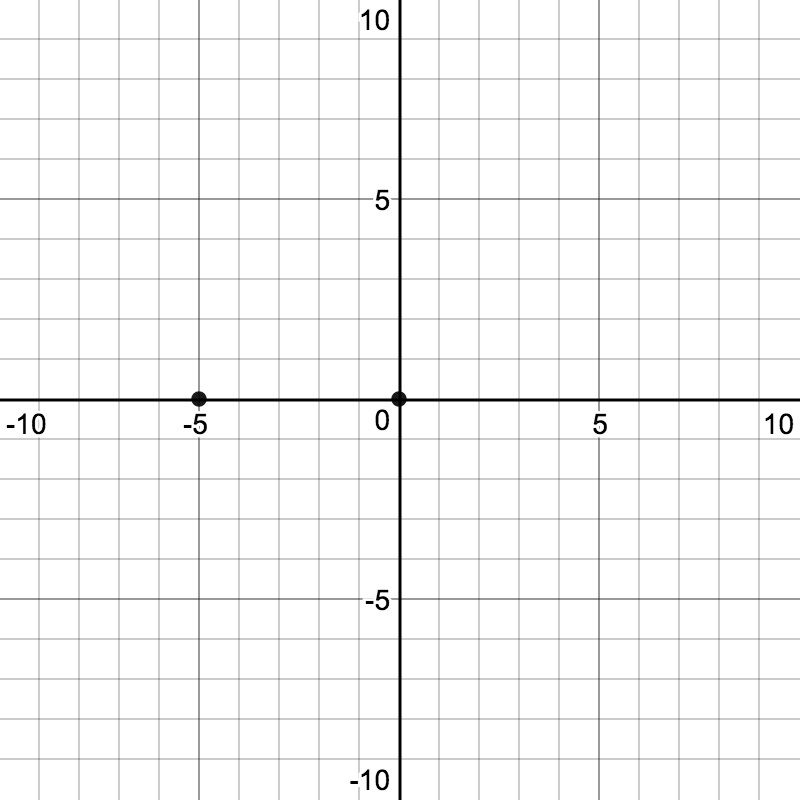## Problem Set

?

The following resources include problems and activities aligned to the objective of the lesson that can be used to create your own problem set.

• Include problems where an “always sometimes never” statement is given, such as “the end behavior is the same for even functions.”

 1.a.     ${y=\frac{1}{2}x^2}$ 2.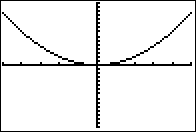b.     ${y=x^3-8}$ 3.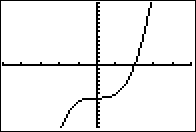c.     ${y=-x^4-x^3+4x+2}$ 4.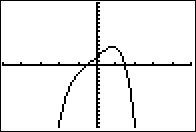d.     ${-3x^3}$ 5.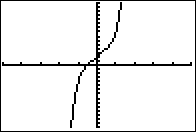e.     ${3x^5-x^3+4x+2}$